# Basic Geometry : Plane Geometry

## Example Questions

### Example Question #51 : Lines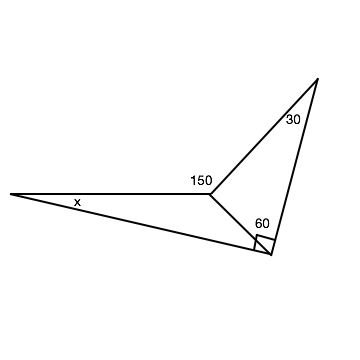In the triangle given, find the missing angle. (Triangles are not drawn to scale.)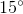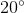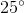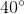Explanation:

To find X, we must first find the other two angles that are inside the triangle with the angle X.

The other acute angle is obtainable because we know it is part of a 90 degree angle, with a line at 60 degrees splitting it, leaving it with 30 degrees for that angle.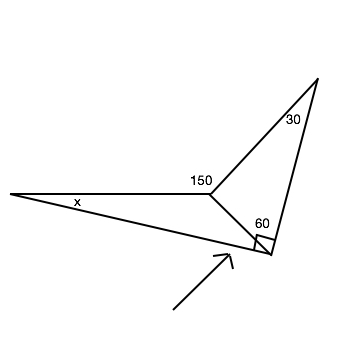So now that we have one, we need to find the other attainable angle inside the triangle with X in it. The central angles will all equal 360 degrees. We know that one of the angles is 150 degrees, but we are not given the other two. The angle where the right arrow is pointing is attainable because we know the other two angles inside that triangle. 180 degrees minus 30 degrees minus 60 degrees leaves us with 90 degrees. Now, after subtracting 360 degrees from the two angles in the interior (150 degrees and 90 degrees), we find that the missing angle is 120 degrees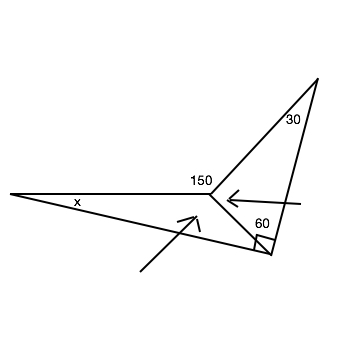Now that we have the two angles needed to find x, the last step is to actually find x.

180 degrees minus 30 degrees minus 120 degrees equals 30 degrees.

X=30 degrees

### Example Question #1581 : Plane Geometry

What angle does the line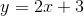create with the y-axis?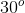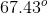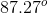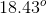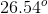Explanation:

The line intersects the y-axis at y = 3. The slope of the line is 2, which means that from y = 3 the next point on the graph is up 2 and over 1. This creates a tiny triangle with the side length adjacent to the angle, on the y-axis, has length 2, and the side length opposite the angle, connecting the y-axis to the graph, has length 1. To find the angle, use tangent: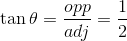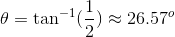### Example Question #1582 : Plane Geometry

What is the measure of the angle formed by the x-axis and the line?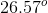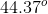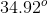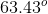Explanation:

The slope of the line is 2, so from the point the graph intersects the x-axis, the graph goes up 2 over 1. Just focusing on the point on the x-axis and the point 2 up and 1 to the right creates a small triangle. The side length adjacent to the angle we're looking for, on the x-axis, is 1, and the side length opposite the angle we're looking for, connecting the graph to the x-axis, has length 2. Use tangent to solve: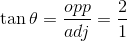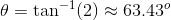### Example Question #54 : Lines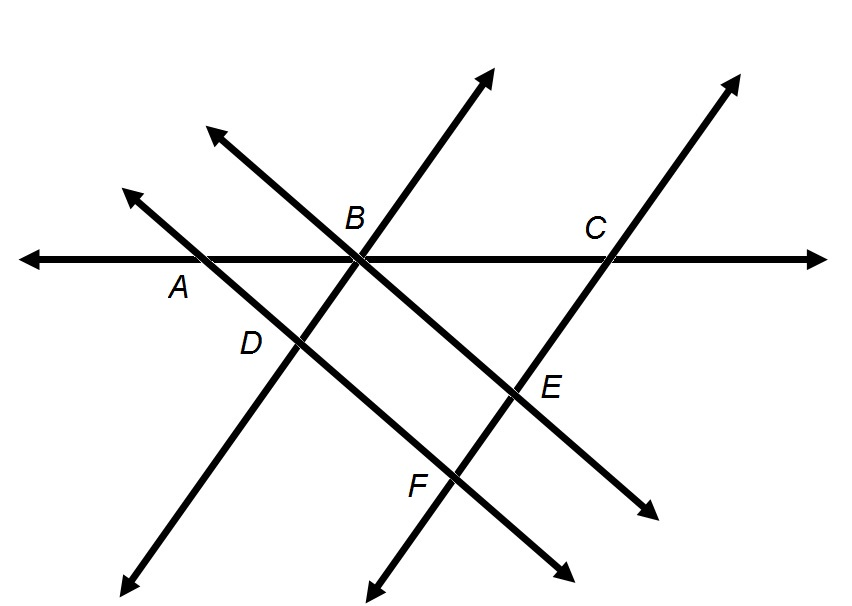Refer to the above diagram.

True or false: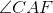can also be called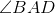.

True

False

True

Explanation:is defined to be the union of rays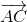and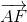, the rays that have endpointand pass through, respectively,and. In the diagram below, the two rays - and, consequently, the angle - are indicated in red.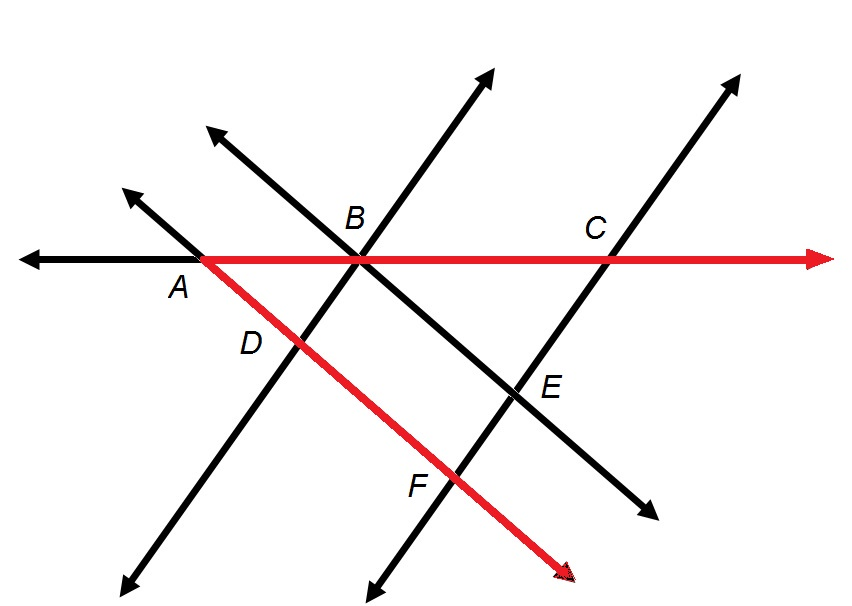andalso pass through, respectively,and, and can be called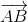and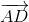. This makesa valid name for the angle.

### Example Question #55 : LinesRefer to the above diagram.

True or false:can also be called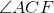.

False

True

False

Explanation:

If an angle is named with three letters, the middle letter is always the vertex of the angle. Therefore,has its vertex at Point, and any three-letter name for this angle must haveas its middle letter. Therefore,cannot be a valid alternative name for the angle.

### Example Question #56 : Lines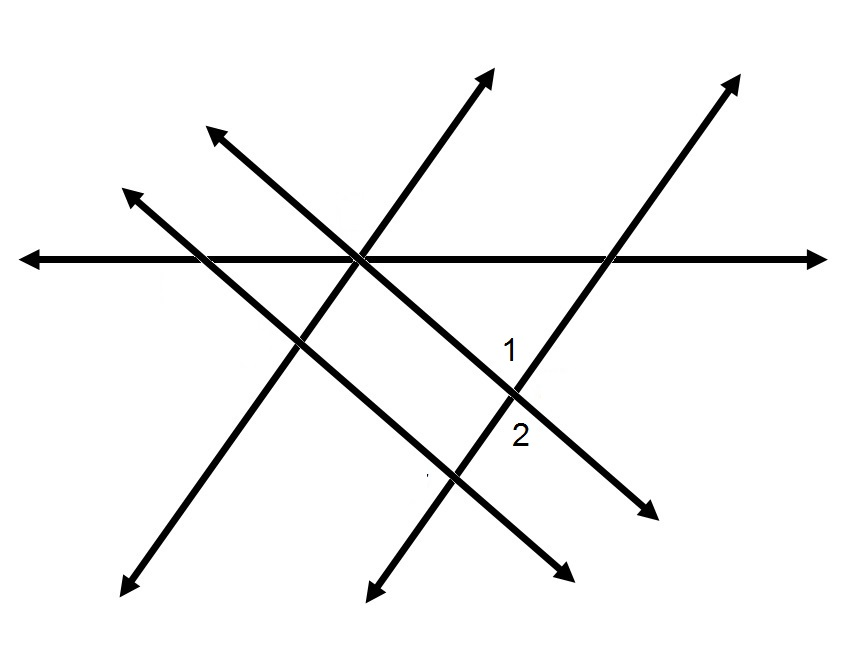Refer to the above diagram.

True or false: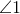and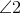comprise a pair of vertical angles.

True

False

True

Explanation:

By definition, two angles comprise a pair of vertical angles if

(1) they have the same vertex; and

(2) the union of the two angles is exactly a pair of intersecting lines.

In the figure below,andare marked in green and red, respectively: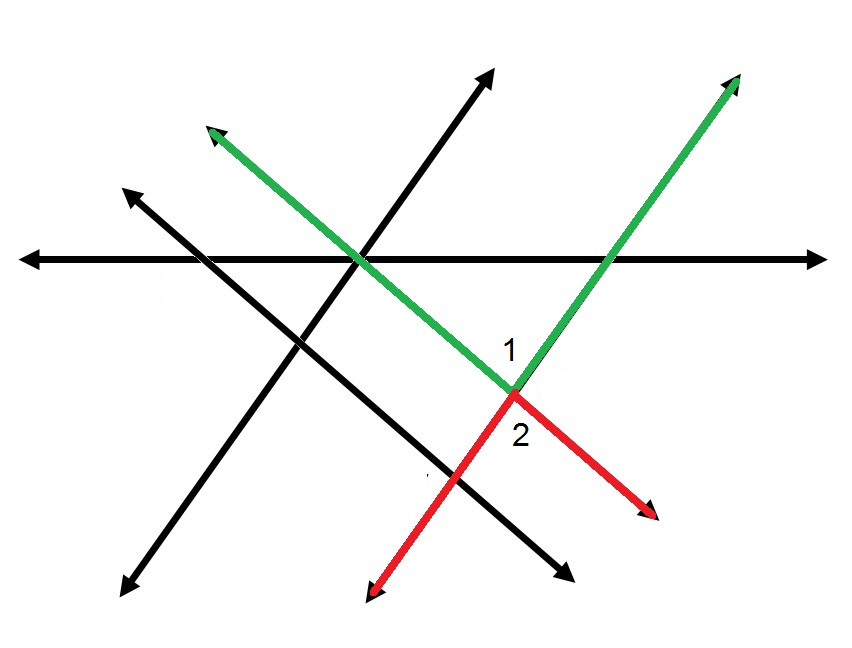The two angles have the same vertex, and their union is a pair of intersecting lines. Therefore, the two angles are indeed a vertical pair.

### Example Question #307 : Coordinate Geometry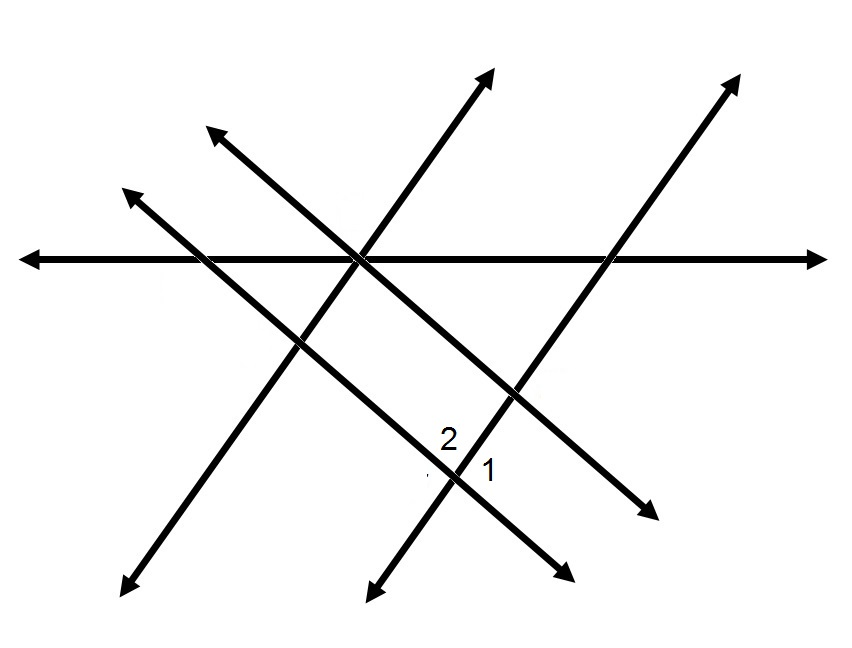Refer to the above diagram.

True or false:andcomprise a pair of vertical angles.

True

False

False

Explanation:

By definition, two angles comprise a pair of vertical angles if

(1) they have the same vertex; and

(2) the union of the two angles is exactly a pair of intersecting lines.

In the figure below,andare marked in green and red, respectively: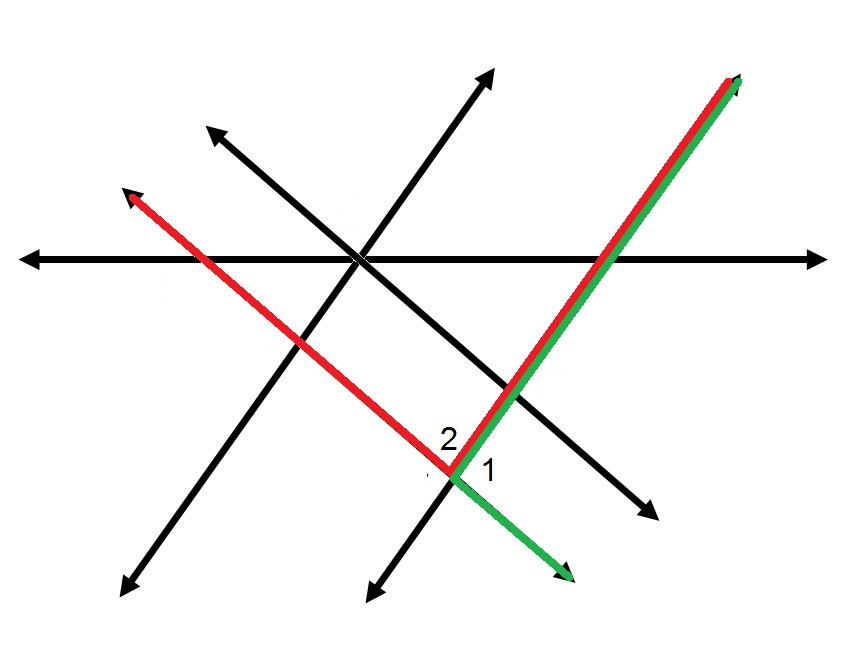While the two angles have the same vertex, their union is not a pair of intersecting lines. The two angles are not a vertical pair.

### Example Question #57 : Lines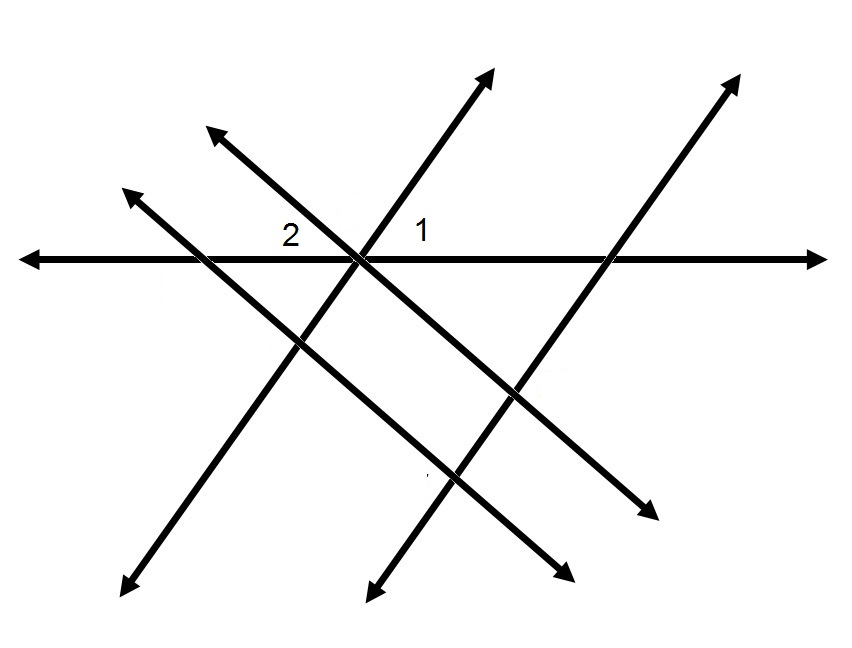Refer to the above diagram.

True or false:andcomprise a pair of vertical angles.

False

True

False

Explanation:

By definition, two angles comprise a pair of vertical angles if

(1) they have the same vertex; and

(2) the union of the two angles is exactly a pair of intersecting lines.

In the figure below,andare marked in green and red, respectively: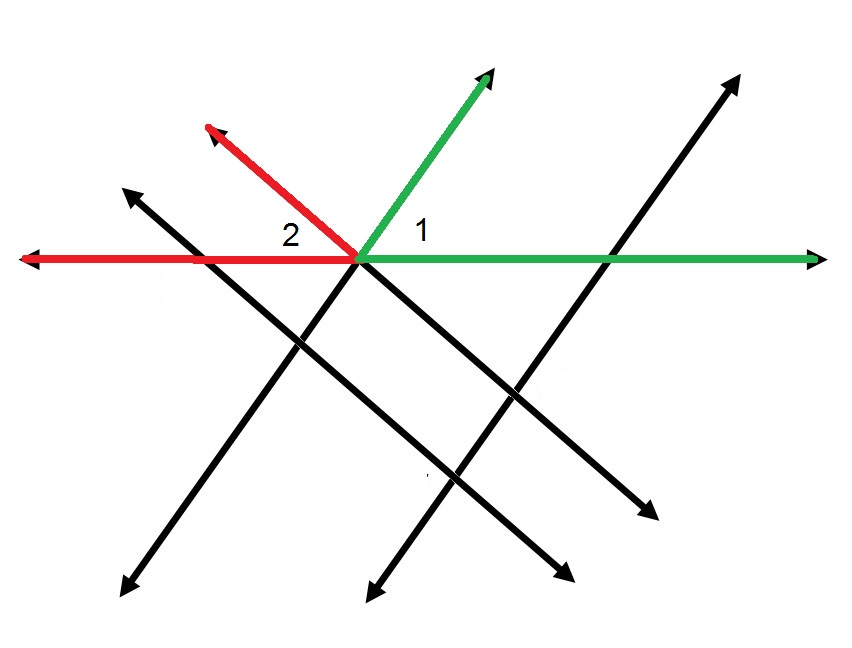While the two angles have the same vertex, their union is not a pair of intersecting lines. The two angles are not a vertical pair.

### Example Question #41 : How To Find An Angle Of A LineRefer to the above diagram.

True or false:andcomprise a linear pair.

False

True

False

Explanation:

By definition, two angles form a linear pair if and only if

(1) they have the same vertex;

(2) they share a side; and,

(3) their interiors have no points in common.

In the figure below,andare marked in green and red, respectively:The two angles have the same vertex and share no interior points. However, they do not share a side. Therefore, they do not comprise a linear pair.

### Example Question #1583 : Plane GeometryRefer to the above diagram.

True or false:andcomprise a linear pair.

True

False

False

Explanation:

By definition, two angles form a linear pair if and only if

(1) they have the same vertex;

(2) they share a side; and,

(3) their interiors have no points in common.

In the figure below,andare marked in green and red, respectively:The two angles have the same vertex and share no interior points. However, they do not share a side. Therefore, they do not comprise a linear pair.

### All Basic Geometry Resources